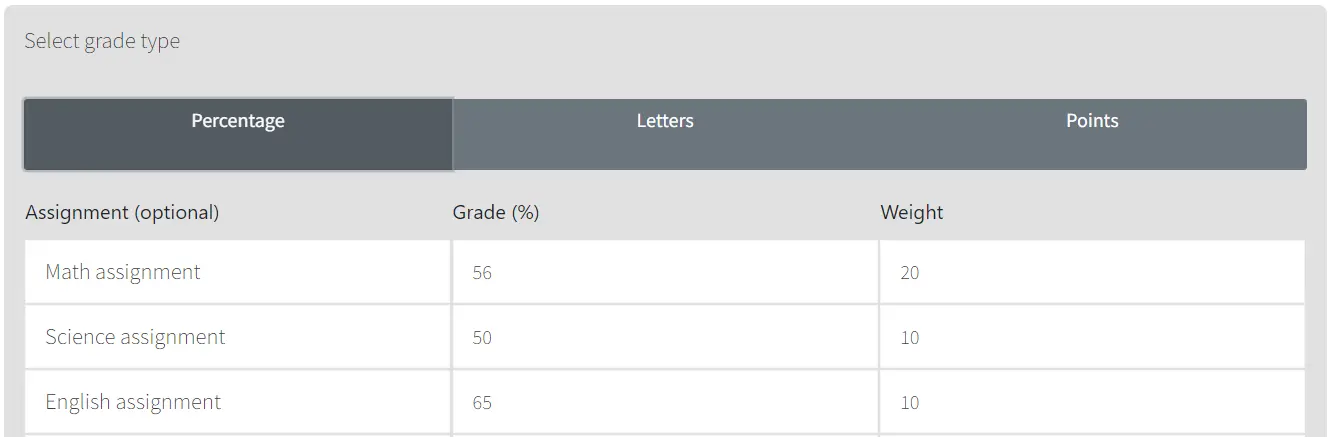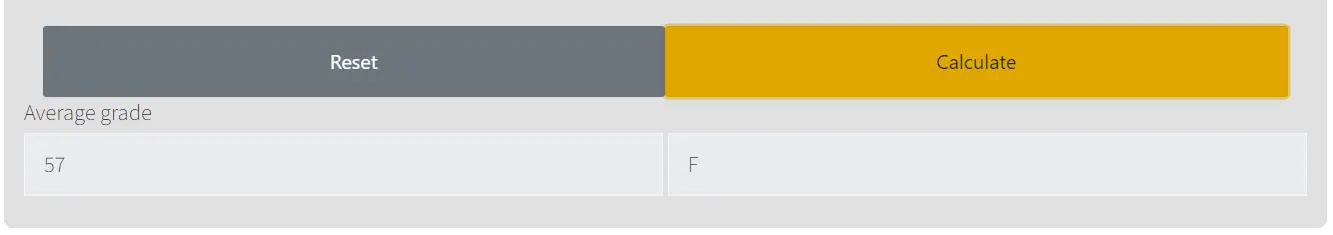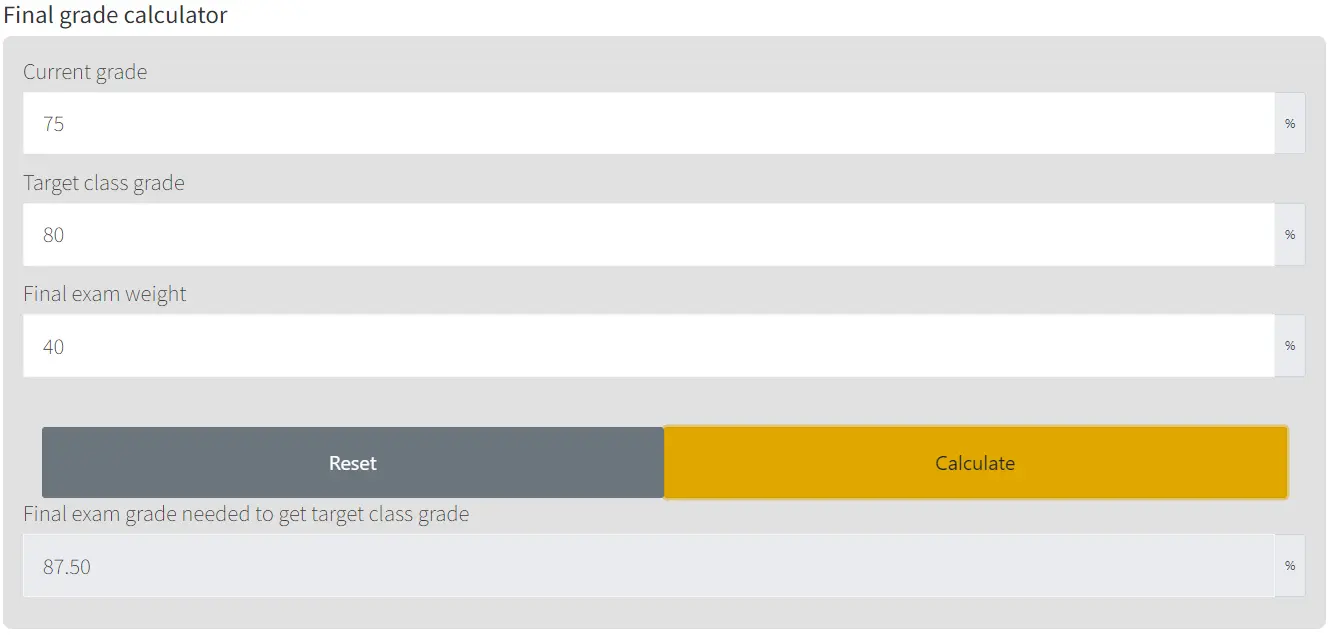• Our grade calculator is the most sophisticated and comprehensive grade calculator online.
• Also has final grade calculation feature.

 Assignment (optional) Grade (letter) Grade (%) Weight (%) -- A+ A A- B+ B B- C+ C C- D+ D D- F -- A+ A A- B+ B B- C+ C C- D+ D D- F -- A+ A A- B+ B B- C+ C C- D+ D D- F -- A+ A A- B+ B B- C+ C C- D+ D D- F -- A+ A A- B+ B B- C+ C C- D+ D D- F -- A+ A A- B+ B B- C+ C C- D+ D D- F -- A+ A A- B+ B B- C+ C C- D+ D D- F -- A+ A A- B+ B B- C+ C C- D+ D D- F Total:

%
%
%
%

Our online Grade Calculator is designed to help both teachers and students calculate grades easily. Using this grade calculator, you can grade any assignment, quiz, or test fast and free. For students, this free tool allows them to be aware of where they stand academically and what is required to maintain or improve their grades throughout the course of the school year. You can use the results of your grade calculation to create goals for the remaining tasks.

Type in the assignment or activity name, then enter your grades together with the weights that each will have in computing your total average.Figure 1. Example calculation

When you click the "Calculate" button, you will see your average in the result.Figure 2. Example result

If you want to calculate your final grade, you can use our easy online final grade calculator. Our final grade calculator will determine the mark you need on your final exam in order to achieve a particular grade.